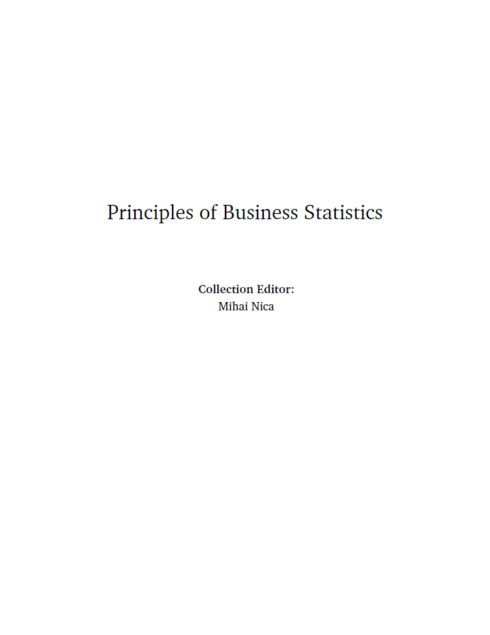(2 reviews)Mihai Nica

Publisher: OpenStax CNX

Language: English

## Conditions of UseAttribution
CC BY

## Reviews

1 Sampling and Data

• 1.1 Sampling and Data: Introduction
• 1.2 Sampling and Data: Statistics
• 1.3 Sampling and Data: Key Terms
• 1.4 Sampling and Data: Data
• 1.5 Sampling and Data: Variation and Critical Evaluation
• 1.6 Sampling and Data: Frequency, Relative Frequency, and Cumulative Frequency

2 Descriptive Statistics

• 2.1 Descriptive Statistics: Introduction
• 2.2 Descriptive Statistics: Displaying Data
• 2.3 Descriptive Statistics: Histogram
• 2.4 Descriptive Statistics: Measuring the Center of the Data
• 2.5 Descriptive Statistics: Skewness and the Mean, Median, and Mode
• 2.6 Descriptive Statistics: Measuring the Spread of the Data

3 The Normal Distribution

• 3.1 Normal Distribution: Introduction
• 3.2 Normal Distribution: Standard Normal Distribution
• 3.3 Normal Distribution: Z-scores
• 3.4 Normal Distribution: Areas to the Left and Right of x
• 3.5 Normal Distribution: Calculations of Probabilities
• 3.6 Central Limit Theorem: Central Limit Theorem for Sample Means
• 3.7 Central Limit Theorem: Using the Central Limit Theorem

4 Confidence Interval

• 4.1 Confidence Intervals: Introduction
• 4.2 Confidence Intervals: Confidence Interval, Single Population Mean, Population Standard Deviation Known, Normal
• 4.3 Confidence Intervals: Confidence Interval, Single Population Mean, Standard Deviation Unknown, Student's-t
• 4.4 Confidence Intervals: Confidence Interval for a Population Proportion

5 Hypothesis Testing

• 5.1 Hypothesis Testing of Single Mean and Single Proportion: Introduction
• 5.2 Hypothesis Testing of Single Mean and Single Proportion: Null and Alternate Hypotheses
• 5.3 Hypothesis Testing of Single Mean and Single Proportion: Using the Sample to Test the Null Hypothesis
• 5.4 Hypothesis Testing of Single Mean and Single Proportion: Decision and Conclusion

6 Linear Regression and Correlation

• 6.1 Linear Regression and Correlation: Introduction
• 6.2 Linear Regression and Correlation: Linear Equations
• 6.3 Linear Regression and Correlation: Slope and Y-Intercept of a Linear Equation
• 6.4 Linear Regression and Correlation: Scatter Plots
• 6.5 Linear Regression and Correlation: The Regression Equation
• 6.6 Linear Regression and Correlation: Correlation Coefficient and Coefficient of Determination
• 6.7 Linear Regression and Correlation: Testing the Significance of the Correlation Coefficient
• 6.8 Linear Regression and Correlation: Prediction

## Ancillary Material

• Submit ancillary resource